# Pandas – GroupBy One Column and Get Mean, Min, and Max values

• Last Updated : 25 Aug, 2020

We can use Groupby function to split dataframe into groups and apply different operations on it. One of them is Aggregation. Aggregation i.e. computing statistical parameters for each group created example – mean, min, max, or sums.

Let’s have a look at how we can group a dataframe by one column and get their mean, min, and max values.

Example 1:

 `import` `pandas as pd`` ` ` ` `# creating a dataframe``df ``=` `pd.DataFrame([(``'Bike'``, ``'Kawasaki'``, ``186``),``                   ``(``'Bike'``, ``'Ducati Panigale'``, ``202``),``                   ``(``'Car'``, ``'Bugatti Chiron'``, ``304``), ``                   ``(``'Car'``, ``'Jaguar XJ220'``, ``210``),``                   ``(``'Bike'``, ``'Lightning LS-218'``, ``218``), ``                   ``(``'Car'``, ``'Hennessey Venom GT'``, ``270``),``                   ``(``'Bike'``, ``'BMW S1000RR'``, ``188``)],``                  ``columns ``=``(``'Type'``, ``'Name'``, ``'top_speed(mph)'``))`` ` `df`

Output :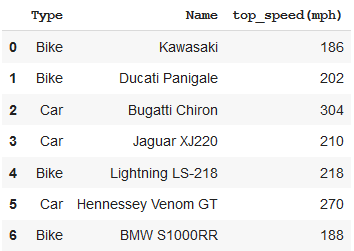Finding mean, min and max values.

 `# using groupby function with aggregation``# to get mean, min and max values``result ``=` `df.groupby(``'Type'``).agg({``'top_speed(mph)'``: [``'mean'``, ``'min'``, ``'max'``]})`` ` `print``(``"Mean, min, and max values of Top Speed grouped by Vehicle Type"``)``print``(result)`

Output :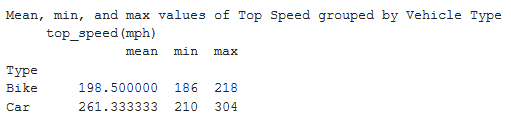Example 2:

 `import` `pandas as pd`` ` ` ` `# creating a dataframe``sales_data ``=` `pd.DataFrame({``'customer_id'``:[``3005``, ``3001``, ``3002``, ``3009``, ``3005``, ``3007``,``               ``3002``, ``3004``, ``3009``, ``3008``, ``3003``, ``3002``],``     ` `'salesman_id'``: [``102``, ``105``, ``101``, ``103``, ``102``, ``101``, ``101``,``                ``106``, ``103``, ``102``, ``107``, ``101``],`` ` `'purchase_amt'``:[``1500``, ``2700``, ``1525``, ``1100``, ``948``, ``2400``,``                ``5700``, ``2000``, ``1280``, ``2500``, ``750``, ``5050``]})`` ` `sales_data`

Output :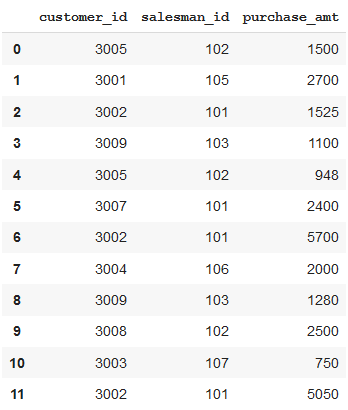Finding mean, min and max values.

 `# using groupby function with aggregation ``# to get mean, min and max values``result ``=` `sales_data.groupby(``'salesman_id'``).agg({``'purchase_amt'``: [``'mean'``, ``'min'``, ``'max'``]})`` ` `print``(``"Mean, min, and max values of Purchase Amount grouped by Salesman id"``)``print``(result)`

Output :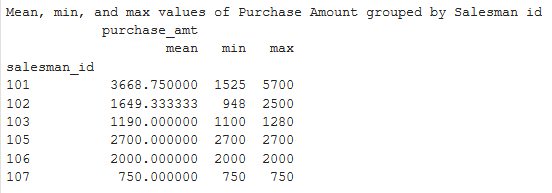Example 3:

 `import` `pandas as pd`` ` ` ` `# creating a dataframe``df ``=` `pd.DataFrame({``"Team"``: [``"Radisson"``, ``"Radisson"``, ``"Gladiators"``,``                            ``"Blues"``, ``"Gladiators"``, ``"Blues"``, ``                            ``"Gladiators"``, ``"Gladiators"``, ``"Blues"``, ``                            ``"Blues"``, ``"Radisson"``, ``"Radisson"``],``                    ` `        ``"Position"``: [``"Player"``, ``"Extras"``, ``"Player"``, ``"Extras"``,``                     ``"Extras"``, ``"Player"``, ``"Player"``, ``"Player"``,``                     ``"Extras"``, ``"Player"``, ``"Player"``, ``"Extras"``],``                    ` `        ``"Age"``: [``22``, ``24``, ``21``, ``29``, ``32``, ``20``, ``21``, ``23``, ``30``, ``26``, ``20``, ``31``]})``df`

Output :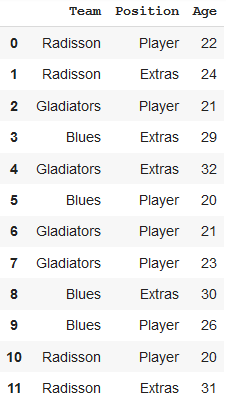Finding mean, min and max values.

 `# using groupby function with aggregation ``# to get mean, min and max values``result ``=` `df.groupby(``'Team'``).agg({``'Age'``: [``'mean'``, ``'min'``, ``'max'``]})`` ` `print``(``"Mean, min, and max values of Age grouped by Team"``)``print``(result)`

Output :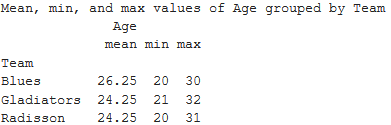My Personal Notes arrow_drop_up